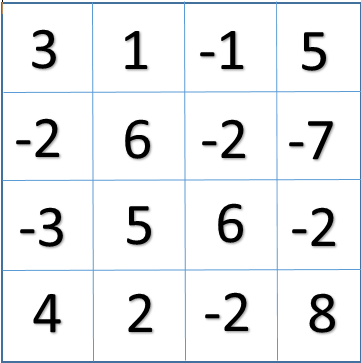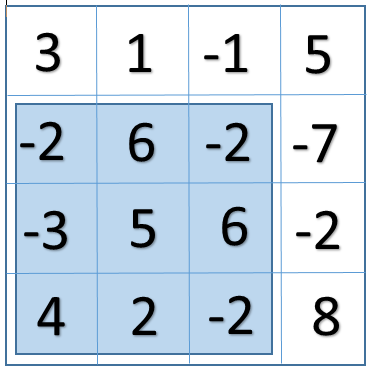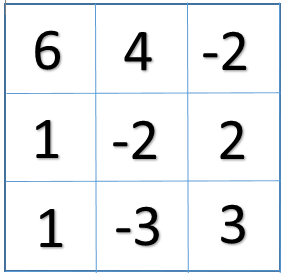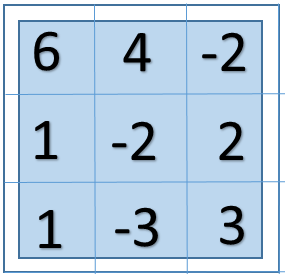×

Search anything:

# Largest rectangular sub matrix having sum divisible by k

#### Algorithms Dynamic Programming (DP)Get this book -> Problems on Array: For Interviews and Competitive Programming

Reading time: 35 minutes | Coding time: 10 minutes

You are given a N X N matrix consisting of some integral values in each cell.The task is to find the largest area of a rectangular sub-matrix such that the total sum of all the elements in that area of the submatrix is divisible by a number k.

## For example if our 4 X 4 matrix is:and if the value of k = 2.
In this case the largest area of a rectangular sub-matrix having sum divisible by 2 is:So basically we have found a submatrix(the one highlighted in blue)which has the maximum area of 9(3 * 3).The submatrix has the top left corner at (1,0) and the bottom right corner at (3,2).The total sum of all the elements of the submatrix is 14 (-2+6-2-3+5+6+4+2-2), which is divisible by 2(which is the value of k in this case).

Input format

We have to input the value of N (4 in the above example), also we will have to input the value of k (2 in above example).The matrix will also be taken as the input.

Output format

Maximum area: 9
Co-ordinate of top left-corner: (1,0)
Co-ordinate of bottom-right corner: (3,2)

## Method:

We will solve the problem by applying the Dynamic Programming approach.

Step 1: One by one we will fix the left and right index for all the possible column in the matrix. For eg: If our N=5 then we will check for index pairs: [ (0,0) (0,1) (0,2) (0,3) (1,1) (1,2) (1,3) .....(3,3) (3,4) (4,4) ]. Now for each left and right column pair we will find the top and bottom indexes of the row such that the sum of the elements in that particular area is divisible by k.

Step 2: For finding the top and bottom indexes of the row we will compute the sum of the elements of each row starting from the left column index and ending at the right column index. After this we will store the sum of every ith row in a temporary array, sum[]; so basically the sum[i] will denote the sum of the elements from left to right for ith row.

Step 3: After this we will apply an algorithm on the sum[] array such that it will find the longest subarray having the sum divisible by k.

Step 4: Whenever we get a subarray of a sum divisible by k then we will update the value of the maxArea (if maxArea< current area) and also the left and right column indexes, and top and bottom row indexes.

## Pseudocode:

``````START
1. Consider all the left and right indexes for every column pair.
2. Store the sum of every row within the left and right indexes in sum[].
3. Follow the steps to find the longest subarray for sum[] which has sum of elements divisible by k.
4. Create a mod[] array where mod[i] stores [(sum+sum...sum[i])%k].
5. Create a map which maps element of mod[] to the element's index of first occurence in mod[].
6. Linearly traverse mod[] from 0 to n and check the following conditions.
7. If mod[i]==0 then update lenMax=(i+1).
8. Else if mod[i] is not present in our map then map mod[i] to i.
9. Else take the value which is mapped for mod[i] and check if lenMax<(i-value).If it is true then update lenMax=(i-value).
END
``````

## Implementation in C++:

``````#include<bits/stdc++.h>
using namespace std;

#define MAX 100

vector<int>LongestSubarrayDivSumK(int sum[], int n, int k);

void LargestRectangle(int mat[MAX][MAX], int n, int k)
{
//Initializing all the variables

int AreaMaximum= 0;
int left,right,i;
int LeftIndex,RightIndex,TopIndex,BottomIndex;
int sum[n];

// vector gives the top and bottom indexes of the rows

vector<int>startAndFinish;

//the start for the left column index

for(left=0; left<n; left++)
{
//Assigning all the values of sum[] to 0

for(i=0;i<n;i++)
sum[i]=0;

//end of the column index
for(right=left; right<n; right++)
{
//calculating the sum for every row

for(i=0;i<n;i++)
{
sum[i]=sum[i]+ mat[i][right];
}

//getting the top and bottom value in startAndFinish vector

startAndFinish= LongestSubarrayDivSumK(sum , n , k);

//the first element of the startAndFinish vector is the TopIndex
//the second element of the startAndFinish vector is the BottomIndex
//Checking for the maximum area

if (AreaMaximum < ((right - left + 1) * (startAndFinish -startAndFinish + 1)))
{
LeftIndex = left; RightIndex = right;
TopIndex = startAndFinish; BottomIndex = startAndFinish;
AreaMaximum = (right - left + 1) * (startAndFinish - startAndFinish + 1);
}
}
}
//Printing the results

cout<<"Maximum Area : "<<AreaMaximum<<endl;
cout<<"Top-left corner : "<<"( "<<TopIndex<<" , ";
cout<<LeftIndex<<" )"<<endl;
cout<<"Bottom-right corner : "<<"( "<<BottomIndex<<" , ";
cout<<RightIndex<<" ) ";
}

vector<int>LongestSubarrayDivSumK(int sum[], int n, int k)
{
int mod[n];
vector<int>StartAndFinish(2);
int presentSum=0;
int LenMax=0;

map<int, int>mp;
int i,j;

//Calculating the value for mod[i] based on the current sum

for(i=0;i<n;i++)
{
presentSum=presentSum+sum[i];
//assigning values to mod[]
mod[i]=presentSum % k;
//for handling negative values
mod[i]=(mod[i]+k)%k;
}

//Checking the conditions for longest subarray

for(i=0;i<n;i++)
{
//if the length is starting from 0 then it has to be max

if(mod[i]==0)
{
StartAndFinish=0;
StartAndFinish=i;
LenMax=i+1;
}

//if mod[i] is present in our map

else if(mp.find(mod[i])!= mp.end())
{
//Checking the max length so far

if(LenMax < (i-mp[mod[i]]))
{
StartAndFinish=mp[mod[i]]+1;
StartAndFinish=i;
LenMax=i-mp[mod[i]];
}
}
//if mod[i] is not present in our map

else
{
mp[mod[i]]=i;
}
}
return StartAndFinish;
}
int main()
{
cout<<"Enter the value for N: "<<endl;
int N;
cin>>N;
cout<<"Enter the value for k : "<<endl;
int k;
cin>>k;
cout<<"Enter your N X N matrix :"<<endl;
int i,j;
int mat[MAX][MAX];
for(i=0;i<N;i++)
{
for(j=0;j<N;j++)
{
cin>>mat[i][j];
}
}
LargestRectangle(mat, N, k);
return 0;
}
``````

## Explanation with an example:

For eg if for N=3 and k=2 our matrix is:So one by one we will consider left and right column indexes :(0,0) (0,1) (0,2) (1,1) (1,2) (2,2)

When we will calculate the sum[] array for (0,2) indexes:
sum[]={8, 1, 1}

So when we apply the above algorithmn to find the longest subarray in sum[] array, we find out that the the complete array itself is the longest subarray having sum divisible by 2(value of k in this case).
Hence we arrive on the conclusion that the largest rectangular sub matrix having sum divisible by k is:Maximum Area : 9
Top-left corner : ( 0 , 0 )
Bottom-right corner : ( 2 , 2 )

## Complexity Analysis:

Time complexity: O(n^3)
Space complexity (Auxillary Space): O(n)

## Applications:

1. The above code can be used to find the maximum area of the rectangular submatrix such that the sum of the elements is divisible by k.

2. With a little modification in the code we can also find all the possible rectangular submatrix with the largest area and having the sum of the elements divisible by k.

## Question

You are given a N X N matrix and you have to find out the largest area of rectangular submatrix such that the sum of all the elements in that submatrix is divisible by an integer k.

Input:
Enter the value for N: .........
Enter the value for k: .........
Enter your N X N matrix: .........

Output:
Maximum Area:........
Top-left corner:.......
Bottom-right corner:.......